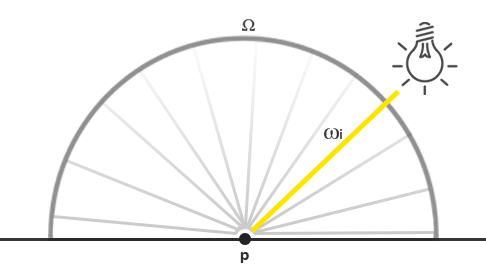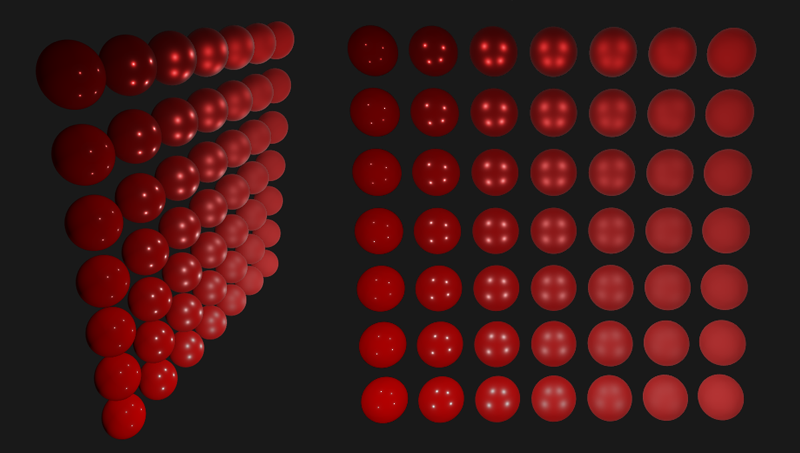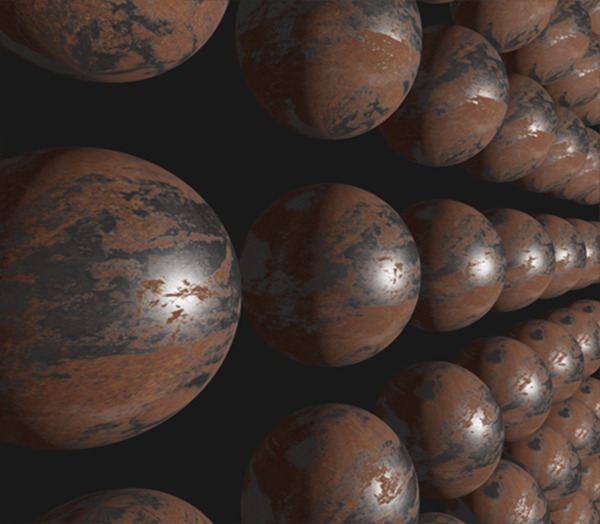# 光照

Note

Importantvec3  lightColor  = vec3(23.47, 21.31, 20.79);
vec3  wi          = normalize(lightPos - fragPos);
float cosTheta    = max(dot(N, Wi), 0.0);
float attenuation = calculateAttenuation(fragPos, lightPos);
float radiance    = lightColor * attenuation * cosTheta;


Important

# 一个PBR表面模型

#version 330 core
out vec4 FragColor;
in vec2 TexCoords;
in vec3 WorldPos;
in vec3 Normal;

uniform vec3 camPos;

uniform vec3  albedo;
uniform float metallic;
uniform float roughness;
uniform float ao;


void main()
{
vec3 N = normalize(Normal);
vec3 V = normalize(camPos - WorldPos);
[...]
}


## 直接光照明

vec3 Lo = vec3(0.0);
for(int i = 0; i < 4; ++i)
{
vec3 L = normalize(lightPositions[i] - WorldPos);
vec3 H = normalize(V + L);

float distance    = length(lightPositions[i] - WorldPos);
float attenuation = 1.0 / (distance * distance);
vec3 radiance     = lightColors[i] * attenuation;
[...]


Important

vec3 fresnelSchlick(float cosTheta, vec3 F0)
{
return F0 + (1.0 - F0) * pow(1.0 - cosTheta, 5.0);
}


vec3 F0 = vec3(0.04);
F0      = mix(F0, albedo, metallic);
vec3 F  = fresnelSchlick(max(dot(H, V), 0.0), F0);


float DistributionGGX(vec3 N, vec3 H, float roughness)
{
float a      = roughness*roughness;
float a2     = a*a;
float NdotH  = max(dot(N, H), 0.0);
float NdotH2 = NdotH*NdotH;

float nom   = a2;
float denom = (NdotH2 * (a2 - 1.0) + 1.0);
denom = PI * denom * denom;

return nom / denom;
}

float GeometrySchlickGGX(float NdotV, float roughness)
{
float r = (roughness + 1.0);
float k = (r*r) / 8.0;

float nom   = NdotV;
float denom = NdotV * (1.0 - k) + k;

return nom / denom;
}
float GeometrySmith(vec3 N, vec3 V, vec3 L, float roughness)
{
float NdotV = max(dot(N, V), 0.0);
float NdotL = max(dot(N, L), 0.0);
float ggx2  = GeometrySchlickGGX(NdotV, roughness);
float ggx1  = GeometrySchlickGGX(NdotL, roughness);

return ggx1 * ggx2;
}


float NDF = DistributionGGX(N, H, roughness);
float G   = GeometrySmith(N, V, L, roughness);


vec3 nominator    = NDF * G * F;
float denominator = 4.0 * max(dot(N, V), 0.0) * max(dot(N, L), 0.0) + 0.001;
vec3 specular     = nominator / denominator;


vec3 kS = F;
vec3 kD = vec3(1.0) - kS;

kD *= 1.0 - metallic;


    const float PI = 3.14159265359;

float NdotL = max(dot(N, L), 0.0);
Lo += (kD * albedo / PI + specular) * radiance * NdotL;
}


vec3 ambient = vec3(0.03) * albedo * ao;
vec3 color   = ambient + Lo;


## 线性空间和HDR渲染

color = color / (color + vec3(1.0));
color = pow(color, vec3(1.0/2.2));## 完整的直接光照PBR着色器

#version 330 core
out vec4 FragColor;
in vec2 TexCoords;
in vec3 WorldPos;
in vec3 Normal;

// material parameters
uniform vec3  albedo;
uniform float metallic;
uniform float roughness;
uniform float ao;

// lights
uniform vec3 lightPositions;
uniform vec3 lightColors;

uniform vec3 camPos;

const float PI = 3.14159265359;

float DistributionGGX(vec3 N, vec3 H, float roughness);
float GeometrySchlickGGX(float NdotV, float roughness);
float GeometrySmith(vec3 N, vec3 V, vec3 L, float roughness);
vec3 fresnelSchlickRoughness(float cosTheta, vec3 F0, float roughness);

void main()
{
vec3 N = normalize(Normal);
vec3 V = normalize(camPos - WorldPos);

vec3 F0 = vec3(0.04);
F0 = mix(F0, albedo, metallic);

// reflectance equation
vec3 Lo = vec3(0.0);
for(int i = 0; i < 4; ++i)
{
vec3 L = normalize(lightPositions[i] - WorldPos);
vec3 H = normalize(V + L);
float distance    = length(lightPositions[i] - WorldPos);
float attenuation = 1.0 / (distance * distance);
vec3 radiance     = lightColors[i] * attenuation;

// cook-torrance brdf
float NDF = DistributionGGX(N, H, roughness);
float G   = GeometrySmith(N, V, L, roughness);
vec3 F    = fresnelSchlick(max(dot(H, V), 0.0), F0);

vec3 kS = F;
vec3 kD = vec3(1.0) - kS;
kD *= 1.0 - metallic;

vec3 nominator    = NDF * G * F;
float denominator = 4.0 * max(dot(N, V), 0.0) * max(dot(N, L), 0.0) + 0.001;
vec3 specular     = nominator / denominator;

float NdotL = max(dot(N, L), 0.0);
Lo += (kD * albedo / PI + specular) * radiance * NdotL;
}

vec3 ambient = vec3(0.03) * albedo * ao;
vec3 color = ambient + Lo;

color = color / (color + vec3(1.0));
color = pow(color, vec3(1.0/2.2));

FragColor = vec4(color, 1.0);
}(上述图片)从下往上球体的金属性从0.0变到1.0， 从左到右球体的粗糙度从0.0变到1.0。你可以看到仅仅改变这两个值，显示的效果会发生巨大的改变！

# 带贴图的PBR

[...]
uniform sampler2D albedoMap;
uniform sampler2D normalMap;
uniform sampler2D metallicMap;
uniform sampler2D roughnessMap;
uniform sampler2D aoMap;

void main()
{
vec3 albedo     = pow(texture(albedoMap, TexCoords).rgb, 2.2);
vec3 normal     = getNormalFromNormalMap();
float metallic  = texture(metallicMap, TexCoords).r;
float roughness = texture(roughnessMap, TexCoords).r;
float ao        = texture(aoMap, TexCoords).r;
[...]
}Important

- 首先是球体的生成，主流的球体顶点生成有两种方法，作者源码采用的是UVSphere方法， IcoSpher方法可以参考这里
- 对于贴图的PBR来说，我们需要TBN矩阵做坐标转换(切线空间-> 世界空间 或者 世界空间 -> 切线空间，参考 法线贴图 章节。)。这有两种方法，一种是在片段着色器中使用叉乘计算TBN矩阵(作者采用的方法)；另外一种是在根据顶点预计算TBN然后VAO中传入TBN矩阵，理论上来说后者会比较快(但是比较麻烦)，不过在译者的实际测试中两者速度差距不大。